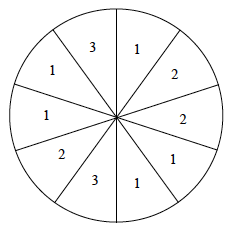### Home > A2C > Chapter 3 > Lesson 3.2.2 > Problem3-116

3-116.

On the spinner at right, each “slice” is the same size. What is the probability that when you spin you will get:

1. The number $1$?

$P=\frac{\text{number of successes}}{\text{total number possible}}$

$P=\frac{1}{2}$

2. The number $2$?

See part (a).

3. The number $3$?

Remember to reduce the fraction.